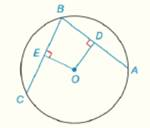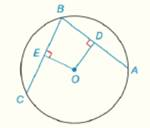Chapter 6.CR, Problem 28CR### Elementary Geometry for College St...

6th Edition
Daniel C. Alexander + 1 other
ISBN: 9781285195698

#### Solutions

Chapter
Section### Elementary Geometry for College St...

6th Edition
Daniel C. Alexander + 1 other
ISBN: 9781285195698
Textbook Problem
1 views

# In Review Exercises 27 to 29, give a proof for each statement. G i v e n :                     ⊙ O with E O - ⊥ B C - ,                                                               D O - ⊥ B A - ,   E O - ≅ O D -             P r o v e :                   B C ^ ≅ B A ^To determine

To Prove: BC^BA^, if O with EO-BC-, DO-BA-, EO-OD-Explanation

Concept:

Theorem 6.1.5:

In a circle (or in congruent circles), congruent chords have congruent minor (major) arcs.

Theorem 6.1.6:

In a circle (or in congruent circles), congruent arcs have congruent chords.

Theorem 6.1.7:

Chords that are at the same distance from the center of a circle are congruent.

Theorem 6.1.8:

Congruent chords are located at the same distance from the center of a circle.

Calculation:

Given that O with EO-BC-, DO-BA-, EO-OD

### Still sussing out bartleby?

Check out a sample textbook solution.

See a sample solution

#### The Solution to Your Study Problems

Bartleby provides explanations to thousands of textbook problems written by our experts, many with advanced degrees!

Get Started

#### Find more solutions based on key concepts